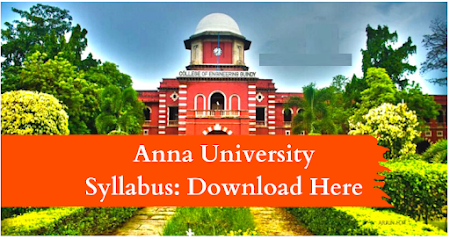### MA8451                      PROBABILITY AND RANDOM PROCESSES              L T P C 4 0 0 4

OBJECTIVES :  To provide necessary basic concepts in probability and random processes for applications such as random signals, linear systems in communication engineering.  To understand the basic concepts of probability, one and two dimensional random variables and to introduce some standard distributions applicable to engineering which can describe real life phenomenon.  To understand the basic concepts of random processes which are widely used in IT fields.  To understand the concept of correlation and spectral densities.  To understand the significance of linear systems with random inputs.

UNIT I   PROBABILITY AND RANDOM VARIABLES                                                             12 Probability – Axioms of probability – Conditional probability – Baye‘s theorem - Discrete and continuous random variables – Moments – Moment generating functions – Binomial, Poisson, Geometric, Uniform, Exponential and Normal distributions.

UNIT II   TWO - DIMENSIONAL RANDOM VARIABLES                                                       12 Joint distributions – Marginal and conditional distributions – Covariance – Correlation and linear regression – Transformation of random variables – Central limit theorem (for independent and identically distributed random variables).

UNIT III   RANDOM PROCESSES                                                                                                12 Classification – Stationary process – Markov process - Markov chain - Poisson process – Random telegraph process.

UNIT IV   CORRELATION AND SPECTRAL DENSITIES                                                        12 Auto correlation functions – Cross correlation functions – Properties – Power spectral density – Cross spectral density – Properties.

UNIT V    LINEAR SYSTEMS WITH RANDOM INPUTS                                                          12 Linear time invariant system – System transfer function – Linear systems with random inputs – Auto correlation and cross correlation functions of input and output.

TOTAL : 60 PERIODS
OUTCOMES: Upon successful completion of the course, students should be able to:  Understand the fundamental knowledge of the concepts of probability and have knowledge of standard distributions which can describe real life phenomenon.  Understand the basic concepts of one and two dimensional random variables and apply in engineering applications.  Apply the concept random processes in engineering disciplines.  Understand and apply the concept of correlation and spectral densities.  The students will have an exposure of various distribution functions and help in acquiring skills in handling situations involving more than one variable. Able to analyze the response of random inputs to linear time invariant systems.

TEXT BOOKS:
1. Ibe, O.C.," Fundamentals of Applied Probability and Random Processes ", 1st Indian Reprint, Elsevier, 2007.
2. Peebles, P.Z., "Probability, Random Variables and Random Signal Principles ", Tata McGraw Hill, 4th Edition, New Delhi, 2002.

REFERENCES:
1. Cooper. G.R., McGillem. C.D., "Probabilistic Methods of Signal and System Analysis", Oxford University Press, New Delhi, 3rd Indian Edition, 2012.
2. Hwei Hsu, "Schaum‘s Outline of Theory and Problems of Probability, Random Variables and Random Processes ", Tata McGraw Hill Edition, New Delhi, 2004.
3. Miller. S.L. and Childers. D.G., ―Probability and Random Processes with Applications to Signal Processing and Communications ", Academic Press, 2004.
4. Stark. H. and Woods. J.W., ―Probability and Random Processes with Applications to Signal Processing ", Pearson Education, Asia, 3rd Edition, 2002.
5. Yates. R.D. and Goodman. D.J., ―Probability and Stochastic Processes", Wiley India Pvt. Ltd., Bangalore, 2nd Edition, 2012